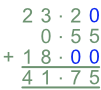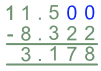Adding and Subtracting Decimals

# Adding and Subtracting Decimals

Basic, GCSE(F),

When adding or subtracting decimals, make sure the decimal points are above each other when working in columns.

Not all numbers may have the same number of digits after the decimal point; add zeroes at the end of numbers to make them all the same length.

When the columns are lined up, the answer will have a decimal point in the correct place.

## Examples

1. Add 23.2, 0.55 and 18.